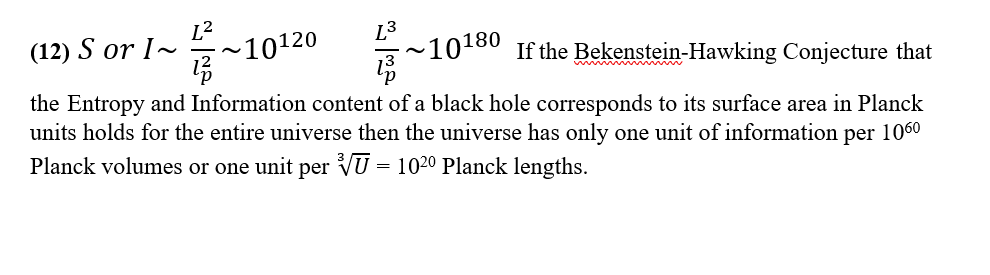## Hypersphere CosmologyAlternative to big-bang

Monday, 22 February 2021 17:00

## Equation 12Equation 12

The Bekenstein-Hawking conjecture arose to explain the behaviour of entropy in a black hole.

It asserts that the entropy does not disappear but becomes preserved by an increase in the surface area of the hole in Planck units if matter falls in.

As the universe itself consists of a black hole in Hypersphere Cosmology then its surface area should represent the total amount of entropy that it contains.

Using the square of the antipode length L over the Planck area to approximate the entropy we obtain an entropy of the order of e120.

If the entropy of a system equates to its information content, then e120 bits of information must suffice for the e180 Planck volumes within the universe.

This implies only one bit of information per e60 Planck volumes or one for each e20 Planck length.

The universe thus has insufficient information to specify anything below this level of its effective pixilation.

See equations 13,14, and 15 for the consequences of this.

None of this supports the Holographic Universe hypothesis. The surface area defines the entropy/information content, but the information is not ‘encoded’ on the surface.

We can obtain a similar result by using the ratios of 3D surface hyper-areas.

The Conservation of Entropy.

Hypersphere Cosmology asserts that the overall entropy of the universe remains constant.

The second law of thermodynamics states that the entropy of a closed system must either increase or remain constant, it can never decrease.
The sky is cold, the stars are hot, plainly the observable entropy remains fairly low, but many of the processes going on around us appear to increase entropy

Now as the limits of observation represent a temporal horizon rather than a temporal boundary, the matter and energy within it has had unbounded time during which it has plainly not achieved thermodynamic equilibrium and maximum entropy.

Thus the universe must have some ongoing processes which increase entropy and some which do the opposite, and between them maintain an overall constant entropy. Gravitation itself may supply the main entropy reversing mechanism.

The principle of never decreasing entropy, the second law of thermodynamics, grew out of the age of steam and observations of the flow of heat in gasses. It takes no account of the attractions between particles. Observe some gas with an uneven distribution of heat or pressure and it will soon homogenise or dissipate. However, a really vast amount of gas will compress itself and ignite as a star. I submit that it be considered as an entropy reversing effect.

If gravity worked repulsively it would fit the simple view of entropy nicely, matter and energy would dissipate. Interestingly the sign of G is formally negative, although we can usually ignore that, but in a sense, gravity reverses the (entropic) arrow of time.

'Entropy increases with time because we measure time in the direction in which entropy increases' - Stephen Hawking.

Equation 10 shows that black holes within the universe will not persist indefinitely.

Neutrons build up as stars burn and create heavier elements but heavier elements breakdown into neutron plasma inside neutron stars which eventually explode. Free neutrons in space decay back into hydrogen.

The recently discovered Giant Gas Halos around nearby galaxies suggest that all galaxies probably have these difficult to observe halos. Such halos, which also contain heavy element dust from exploded stars, may well act as the recycling yards for the stellar parts of the galaxies.

We have no means of determining the ages of black holes and some structures within the universe seem to have an age very close to, or in excess of, the temporal horizon.

It does not seem unlikely that the closed spacetime of the Hyperspherical Universe can maintain a constant leel of entropy.

More in this category: « Equation 13 Equation 11 »
• #### HyperSphere Cosmology +Hypersphere Cosmology. (6)    P J Carroll 28/2/2021   Abstract.
• #### Type 1A supernovae and Hypersphere Cosmology +

Lensing, Redshift and Distance for Type1A Supernovae. All data input from Perlmutter et all, https://arxiv.org/pdf/astro-ph/9812133.pdf The measured Apparent Magnitudes of
• #### Galactic Rotation Curves +

Galactic Rotation Curves. A large discrepancy exists between the rotation curves for disc galaxies expected from a classical or relativistic
• #### Equation 17 +

Equation 17 The Redshift-Distance and the Lensing equations 6 and 16 together yield a derivation for the Antipode length L
• #### Equation 16 +

Equation 16 A stereographic projection can show the effect of projecting an n-sphere into an n-dimensional space. For
• #### Equation 15 +

Equation 15 It may seem odd that whilst the ‘enhanced’ Planck length and Planck time set a lower limit for
• #### Equation 14 +

Equation 14 The universe has an information deficit in the sense that it has only one bit of information/entropy for
• #### Equation 13 +

Equation 13 The universe has an information deficit in the sense that it has only one bit of information/entropy for
• #### Equation 12 +

Equation 12 The Bekenstein-Hawking conjecture arose to explain the behaviour of entropy in a black hole. https://en.wikipedia.org/wiki/Black_hole_thermodynamics#Overview It asserts that
• #### Equation 11 +

Equation 11 The Dirac Large Numbers Hypothesis suggests that a huge dimensionless number, or perhaps just a few huge dimensionless
• #### Equation 10 +

Equation 10 Here we show the modification to Newtonian Dynamics expected in a positively curved spacetime that has a negative
• #### Equation 9 +

Equation 9 Galaxies rotate but they do not rotate in accordance with our standard ideas about gravity. A large discrepancy
• 1
• 2